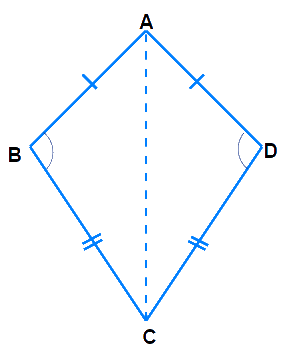# Ex.3.3 Q4 Understanding Quadrilaterals Solution-Ncert Maths Class 8

Go back to  'Ex.3.3'

## Question

Draw a rough figure of a quadrilateral that is not a parallelogram but has exactly two opposite angles of equal measure.

Video Solution
Ex 3.3 | Question 4

## Text Solution

What is Known?

Draw a figure of quadrilateral having two opposite angles of equal measure.

What is Unknown?

$$ABCD$$ is quadrilateral whose opposite angles are equal.

Reasoning:

The opposite angles of a parallelogram are equal.

Steps:In a kite, the angle between unequal sides are equal.

Draw line from $$A$$ to $$C$$ and we will get two triangles with common base $$AC$$.

In $$∆ABC$$ and $$∆ADC$$ we have,

$${\rm{AB}} = {\rm{ AD }},{\rm{ BC}} = {\rm{CD}}$$ ; $$AC$$ is common to both

$$\Delta {\rm{ABC }}\!\!\cong\!\!\Delta {\rm{ADC }}\text{[congruent triangles]}$$

Hence corresponding parts of congruent triangles are equal.

Therefore$$\angle {\rm{B}} = \angle {\rm{D}}$$

However, the quadrilateral $$ABCD$$ is not a parallelogram as the measures of the remaining pair of opposite angles, $$\angle {\rm{A}}$$ and $$\angle {\rm{C}}$$, are not equal. Since they form angle between equal sides.

Learn from the best math teachers and top your exams

• Live one on one classroom and doubt clearing
• Practice worksheets in and after class for conceptual clarity
• Personalized curriculum to keep up with school